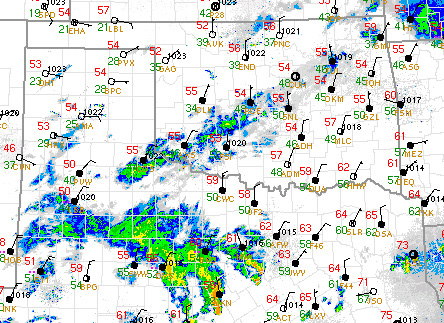[--MAIN HOME--] [--ALL HABYHINTS--] [--FACEBOOK PAGE--]

 WHAT IS THE TEMP AND DEWPOINT?

METEOROLOGIST JEFF HABY

On a surface station plot, typically the temperature and dewpoint are given together. On U.S. surface plots, this data is typically given in degrees Fahrenheit. The temperature is a measurement of the kinetic energy in the air. As air molecules increase in kinetic energy then the temperature goes up. Since each air molecule can move at a somewhat different speed, the temperature is the average kinetic energy. Temperature is also described as the relative hotness of coldness of the air in reference to a particular standard measurement system. The dewpoint is the temperature the air would need to be cooled in order for saturation to occur. For example, if the temperature is 50 F and the dewpoint is 40 F, then the temperature would have to cool from 50 F to 40 F in order for the air to become saturated, assuming a constant moisture content in the air. Once the temperature cools to the dewpoint then the relative humidity is 100% and the air is said to be saturated. The surface plot below shows an example of temperature and dewpoint. The temperature is given in red while the dewpoint is in green. The temperature is always greater than or equal to the dewpoint. The dewpoint will never be greater than the temperature. The closer the temperature and dewpoint are to each other then the closer the air is to being saturated. Notice over northern Texas in the Dallas area that the temperature and dewpoint are only a few degrees from each other. This indicates fairly humid conditions with a high relative humidity since temperatures are mild and the temperature is close to the dewpoint. Notice over the panhandle of Texas that the difference between the temperature and dewpoint is around 20 units of F or more. This indicates drier air and a lower relative humidity. The difference between the temperature and dewpoint is called a dewpoint depression. A dewpoint depression is not an actual temperature but rather the value obtained by taking the temperature and subtracting the dewpoint. Temperature and dewpoint are two basic values that forecasters use to examine the weather situation.